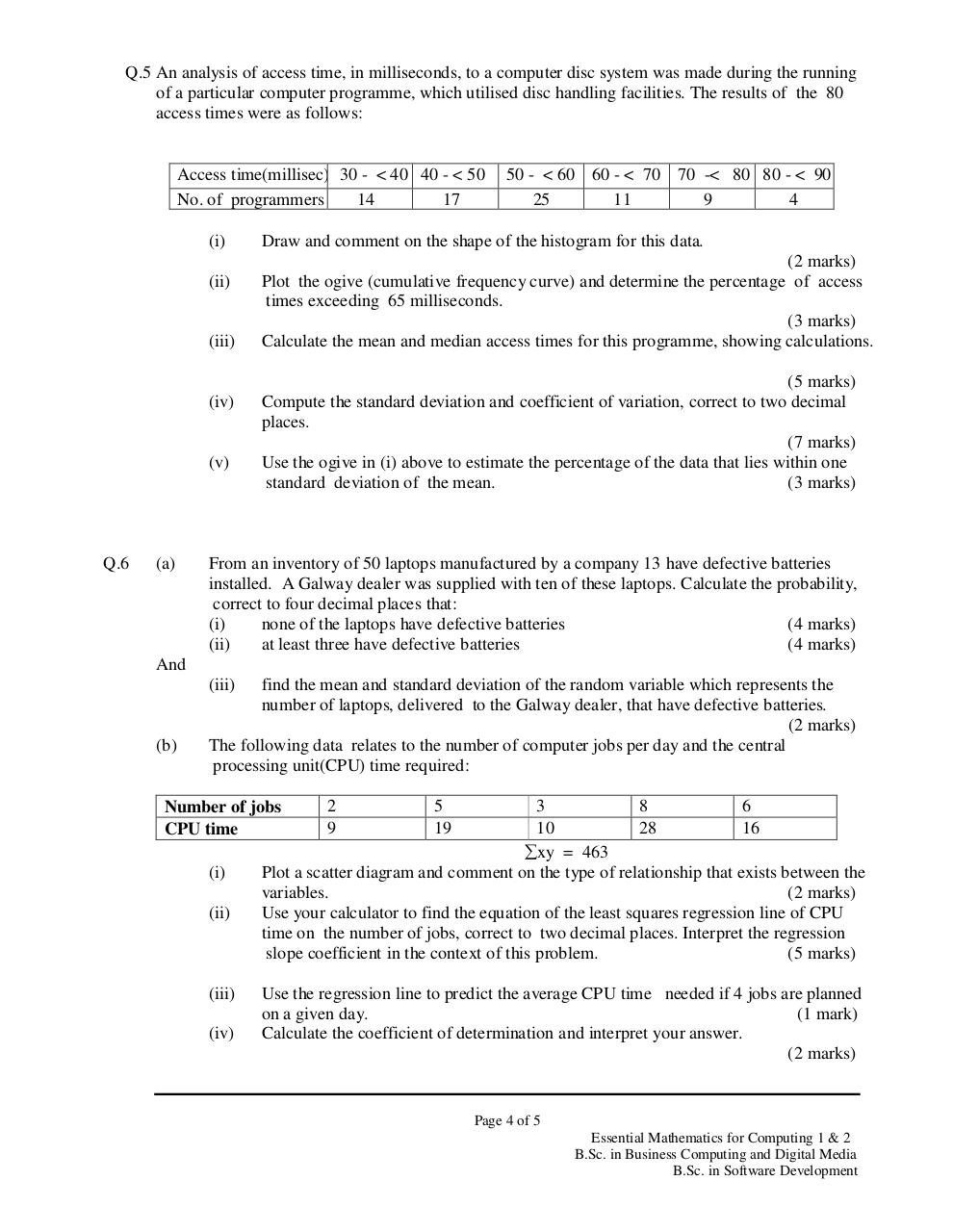# Maths (Summer 2010).PDFPage 1 2 3 4 5 6

#### Text preview

Q.5 An analysis of access time, in milliseconds, to a computer disc system was made during the running
of a particular computer programme, which utilised disc handling facilities. The results of the 80
access times were as follows:

Access time(millisec) 30 - &lt; 40 40 - &lt; 50
No. of programmers
14
17
(i)
(ii)

(iii)

(iv)

(v)

Q.6

(a)

50 - &lt; 60 60 - &lt; 70 70 -&lt; 80 80 - &lt; 90
25
11
9
4

Draw and comment on the shape of the histogram for this data.
(2 marks)
Plot the ogive (cumulative frequency curve) and determine the percentage of access
times exceeding 65 milliseconds.
(3 marks)
Calculate the mean and median access times for this programme, showing calculations.
(5 marks)
Compute the standard deviation and coefficient of variation, correct to two decimal
places.
(7 marks)
Use the ogive in (i) above to estimate the percentage of the data that lies within one
standard deviation of the mean.
(3 marks)

From an inventory of 50 laptops manufactured by a company 13 have defective batteries
installed. A Galway dealer was supplied with ten of these laptops. Calculate the probability,
correct to four decimal places that:
(i)
none of the laptops have defective batteries
(4 marks)
(ii)
at least three have defective batteries
(4 marks)

And
(iii)

(b)

find the mean and standard deviation of the random variable which represents the
number of laptops, delivered to the Galway dealer, that have defective batteries.
(2 marks)
The following data relates to the number of computer jobs per day and the central
processing unit(CPU) time required:
3
8
6
10
28
16
∑xy = 463
Plot a scatter diagram and comment on the type of relationship that exists between the
variables.
(2 marks)
Use your calculator to find the equation of the least squares regression line of CPU
time on the number of jobs, correct to two decimal places. Interpret the regression
slope coefficient in the context of this problem.
(5 marks)

Number of jobs
CPU time
(i)
(ii)

(iii)
(iv)

2
9

5
19

Use the regression line to predict the average CPU time needed if 4 jobs are planned
on a given day.
(1 mark)